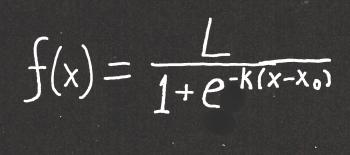Games
Problems
Go Pro!

Wesley asks, "I have been reading your answers to the corona virus questions and came across the term logistic curve. I am interested in the math behind them, and do not see how you can make a logistic curve unless one of two things. One, you take the inverse of an odd degree polynomial or two somehow you can incorporate the last y-value to influence the next x-value. I have come across this problem before and I would like to know if there is an alternate solution that can make a logistic curve an actual function of y in terms of x. If there isn't, thank you anyways for your time and effort."

That's a great question, Wesley. I like your thinking regarding odd degree polynomials. Odd degree polynomials do have an inflection point similar to a logistic curve. The problem with polynomial equations is that they don't have asymptotes. An asymptote is a straight line that the graph approaches without ever touching. A simple example of a graph with asymptotes is xy = 1. That graph gets closer and closer to the x and y axes, but never touches it.

A logistic curve has two horizontal asymptotes - one at the base of the curve, and one at the top. So a polynomial function (even an odd one) won't do the trick. What we need is an exponential function. Here's what you're looking for:This is a messy looking function, but hopefully I can talk you through the concepts fairly simply. First, just in case you (or another reader) doesn't know what "e" is - it's the natural logarithm, which is approximately 2.71828. L, k, and x0 are also constants. The are set values for any particular problem or real-world application.

A "normal" exponential function might look something like this: f(x) = ekx. k controls how quickly the function "blows up" and x is the independent variable. Since we're thinking about virus spread, the independent variable is time - perhaps the number of days that have passed.

The logistic curve has elements of an exponential curve. It's interesting that the "e" is in the denominator, and also that it has a negative exponent - those two features sort of "cancel" each other out, because a negative exponent indicates taking the reciprocal.  But it's the other stuff in the denominator with the e that makes this function's behavior interesting.

First, if x < x0, then the exponent of e is a positive number, and the denominator will be growing larger the further x is from x0. This results in a large denominator, which results in the value of the fraction approaching 0.

On the other hand, if x > x0, then e has a negative exponent, which means it is getting closer and closer to 0. This means the denominator is getting closer and closer to 1, and the function value is getting closer and closer to L.

This gives us our two asymptotes: y = 0 and y = L. Thus, 0 < f(x) < L.

And the point x = x0 is the point at which the function switches from blowing up to leveling off.

TO SUMMARIZE: in the logistic curve, k determines the rate at which the function grows, L represents the maximum value, and x0  represents the point in time at which the graph has its inflection point. In terms of a viral infection, L is the number of people who will be infected, and x0 is the time at which the number of new infections begins decreasing.# Blogs on This SiteReviews and book lists - books we love!The site administrator fields questions from visitors.Like us on Facebook to get updates about new resources